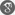##### Cite as:
Karunesh Kumar Singh, Asha Ram Gairola, Deepmala ., Approximation Theorems for q- Analouge of a Linear Positive Operator by A. Lupas, Int. J. Anal. Appl., 12 (1) (2016), 30-37.

#### Abstract

The purpose of the present paper is to introduce \$q-\$ analouge of a sequence of linear and positive operators which was introduced by A. Lupas . First, we estimate moments of the operators and then prove a basic convergence theorem. Next, a local direct approximation theorem is established. Further, we study the rate of convergence and point-wise estimate using the Lipschitz type maximal function.

#### References

1. A. Lupas, The approximation by some positive linear operators. In: proceedings of the International Dortmund meeting on Approximation Theory(M.W. Müller et al., eds.), akademie Verlag, Berlin, 1995, 201-229.2. B. Lenze, On Lipschitz-type maximal functions and their smoothness spaces, Nederl. Akad. Wetensch. Indag. Math. 50(1) (1988), 531-763.3. R.A. DeVore and G. G. Lorentz, Constructive Approximation, Volume 303. Springer-Verlag, Berlin, 19934. A.D. Gadjiev, R. O. Efendiyev, and E. Ibikli, On Korovkin type theorem in the space of locally integrable functions, Czechoslovak Mathematical Journal, 53 (1) (2003), 45-53.5. A.D. Gadjiev, Theorems of the type of P. P. Korovkin’s theorems, Math. Zametki, 20(5) (1976), 7811-7786.6. A.R. Gairola, Deepmala, and L.N. Mishra, Rate of Approximation by Finite Iterates of q-Durrmeyer Operators, Proceedings of the National Academy of Sciences, India Section A: Physical Sciences, 86(2) (2016), 229-234.7. M. A. Ozarslan, O. Duman and H.M. Srivastava, Statistical approximation results for Kantorovich-type operators involving some special polynomials, Math. Comput. Modelling, 48 (2008), 388-401.8. V.N. Mishra, P. Sharma, and L.N. Mishra; On statistical approximation properties of q−Baskakov-Szász-Stancu operators, Journal of Egyptian Mathematical Society, 24 (3) (2016), 396-401.9. H.M. Srivastava, M. Mursaleen, and A. Khan, Generalized equi-statistical convergence of positive linear operators and associated approximation theorems, Math. Comput. Model. 55 (2012), 2040-2051.10. V.N. Mishra, K. Khatri, and L.N. Mishra, Statistical approximation by Kantorovich type Discrete q−Beta operators, Advances in Difference Equations 2013 (2013), Art. ID 345.11. M. Mursaleen, A. Khan, H.M. Srivastava, and K.S. Nisar, Operators constructed by means of q-Lagrange polyno- mials and A-statistical approximation, Appl. Math. Comput. 219 (2013), 6911-6918.12. N.L. Braha, H.M. Srivastava and S.A. Mohiuddine, A Korovkin’s type approximation theorem for periodic functions via the statistical summability of the generalized de la Vall´ ee Poussin mean, Appl. Math. Comput. 228 (2014), 162- 169.13. V.N. Mishra, K. Khatri, L.N. Mishra, and Deepmala; Inverse result in simultaneous approximation by Baskakov- Durrmeyer-Stancu operators, Journal of Inequalities and Applications 2013 (2013), Art. ID 586.14. V.N. Mishra, H.H. Khan, K. Khatri, and L.N. Mishra; Hypergeometric Representation for Baskakov-Durrmeyer- Stancu Type Operators, Bulletin of Mathematical Analysis and Applications, 5 (3) (2013), 18-26.15. V.N. Mishra, K. Khatri, and L.N. Mishra; On Simultaneous Approximation for Baskakov-Durrmeyer-Stancu type operators, Journal of Ultra Scientist of Physical Sciences, 24 (3) (2012), 567-577.16. V.N. Mishra, K. Khatri, and L.N. Mishra, Some approximation properties of q-Baskakov-Beta-Stancu type opera- tors, Journal of Calculus of Variations, 2013 (2013), Article ID 814824.17. A. Wafi, N. Rao, and Deepmala, Approximation properties by generalized-Baskakov-Kantorovich-Stancu type op- erators, Appl. Math. Inf. Sci. Lett., 4 (3) (2016), 1-8.